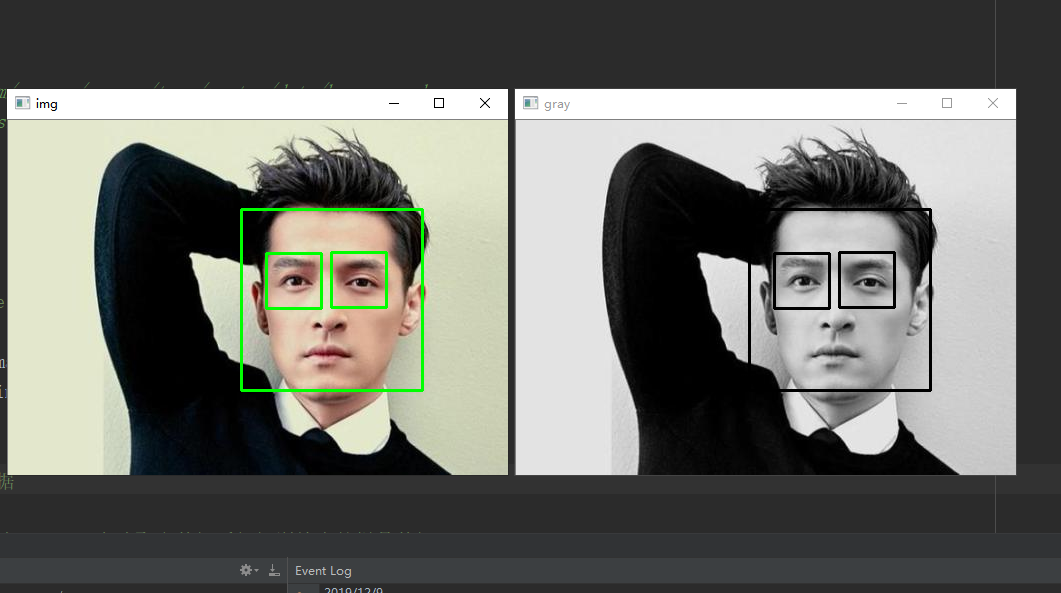﻿﻿﻿﻿ python中用opencv实现人脸识别

### python中用opencv实现人脸识别

• 时间：2019-12-11
• 676人已阅读

```# -*- coding: UTF-8 -*-
"""
opencv实现人脸识别

xml 来源
2、http://www.cnblogs.com/hanson1/p/7105265.html

"""
import cv2

# 待检测的图片路径
imagepath="./img/huge1.jpg"

gray = cv2.cvtColor(image,cv2.COLOR_BGR2GRAY)

'''
# 获取人脸识别训练数据

xml数据下载，

'''
#cv2.namedWindow("img",0);

# 探测人脸
# 根据训练的数据来对新图片进行识别的过程。
gray,
scaleFactor = 1.15,
minNeighbors = 5,
minSize = (5,5),
)
gray,
scaleFactor = 1.15,
minNeighbors = 3,
minSize = (3,3),
)

# 我们可以随意的指定里面参数的值，来达到不同精度下的识别。返回值就是opencv对图片的探测结果的体现。

# 处理人脸探测的结果
print ("发现{0}个人脸!".format(len(faces)))
#标识人脸
for(x,y,w,h) in faces:
cv2.rectangle(image,(x,y),(x+w,y+h),(0,255,0),2)
cv2.rectangle(gray, (x, y), (x + w, y + h), (0, 255, 0), 2)
roi_face = gray[y:y + h, x:x + w]
roi_color = image[y:y + h, x:x + w]
# 框住眼睛 为了减少运算量 把脸部作为输入
roi_face,
scaleFactor = 1.66,
minNeighbors = 6,
minSize = (6,5),
)
for (e_x, e_y, e_w, e_h) in eyes:
cv2.rectangle(roi_color, (e_x, e_y), (e_x + e_w, e_y + e_h), (0, 255, 0), 2)
cv2.rectangle(roi_face, (e_x, e_y), (e_x + e_w, e_y + e_h), (0, 255, 0), 2)

cv2.imshow("img",image)
cv2.imshow("gray",gray)
cv2.waitKey(0)
cv2.destroyAllWindows()```Top